Author: Oscar Cronquist Article last updated on May 21, 2022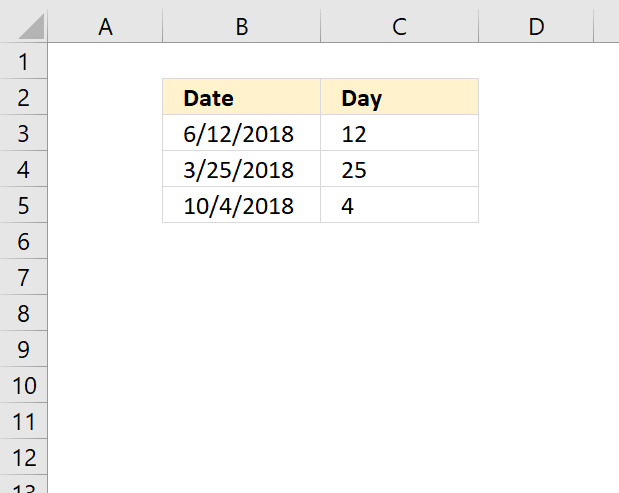The DAY function extracts the day as a number from an Excel date.

## 1. DAY Function Syntax

DAY(serial_number)

## 2. DAY Function Arguments

 serial_number Required. The Excel date value you want to extract the day number from.

Excel dates are actually numbers formatted as dates. January 1, 1900 is serial number 1, and January 1, 2008 is serial number 39448 because it is 39,448 days after January 1, 1900.

## 3. DAY function exampleThe image above demonstrates a formula in cell C3 that extracts the day number from a date, however, the date must be an Excel date.

Formula in cell C3:

=DAY(B3)

To understand the DAY function I need to explain that it needs an Excel date in order to calculate the day properly.  The date in cell B3 is an Excel date meaning it is a number formatted as a date. 1 is 1/1/1900 and 1/1/2000 is 36526 meaning there are 36526 days between the dates.

You can verify this, select a cell containing 1/1/2000 and press CTRL + 1 to open the "Format Cells" dialog box.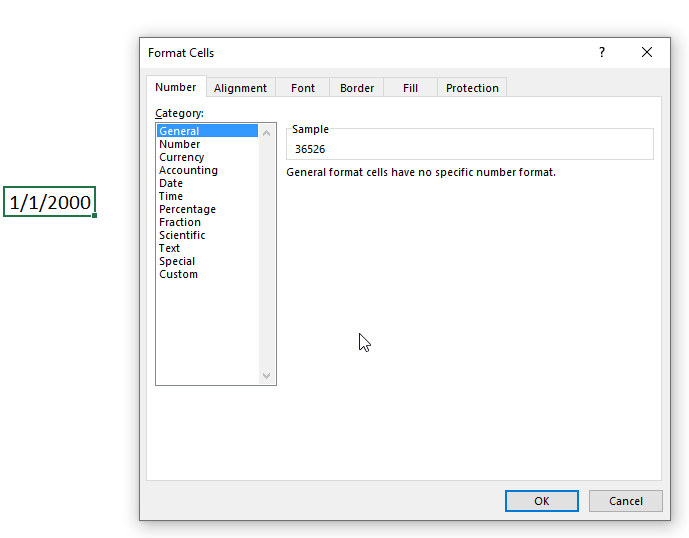## 4. DAY function not working

• Make sure the date is an Excel date.

How do I know the date is recognized as an Excel date?
Check the "Format Cells" dialog box and press with left mouse button on category "General". You should see a number and not a date.

How do I convert a date to an Excel date?
Try using the DATEVALUE function to convert a text date to an Excel date. Read this article if it is not working.

## 5.1 DAY function alternative - TEXT function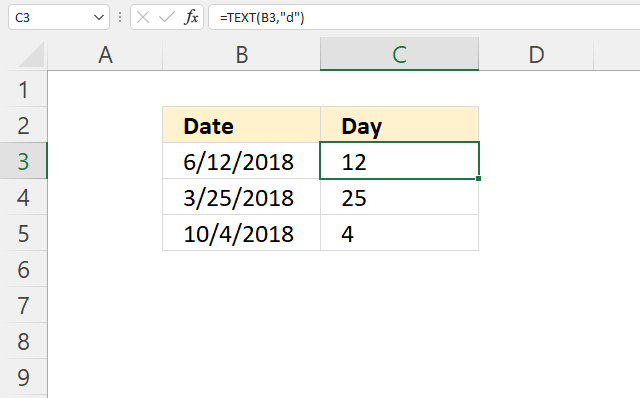Formula in cell C3:

=TEXT(B3,"d")

### 5.1.1 Explaining formula

#### Step 1 - TEXT function

The TEXT function lets you format values.

TEXT(valueformat_text)

value - The string you want to format. You can use a cell reference here or use a text string.

format_text - Formatting code allowing you to change the way, for example, a date or a number is displayed to the Excel user.

#### Step 2 - Populate arguments

The TEXT function has two arguments.

value - B3

format_text - "d"

"d" is an abbreviation for day. A single "d" returns the day like this: 1. A double "dd" returns 01.

TEXT(B3, "d")

becomes

TEXT(43263, "d")

and returns 12.

## 5.2 DAY function alternative - Cell formatting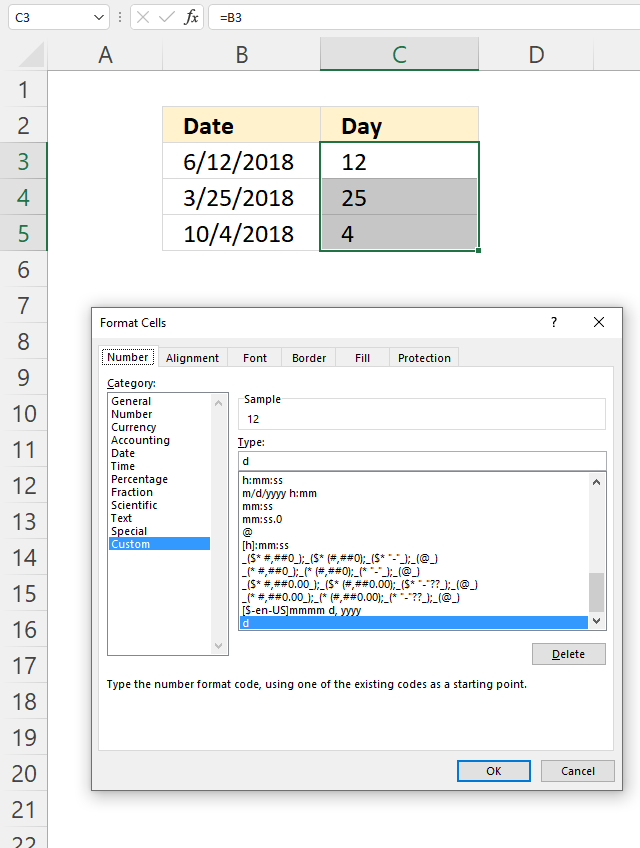You can also show the day number using cell formatting, the image above shows cell formatting applied to cell C5.

How to apply cell formatting:

1. Select cell C3.
2. Press CTRL + 1 to open the "Format Cells" dialog box.
3. Press with left mouse button on "Category" Custom, see the image above.
4. Enter d below Type:
5. Press with left mouse button on the OK button to apply changes.

## 6. Filter dates based on a given day number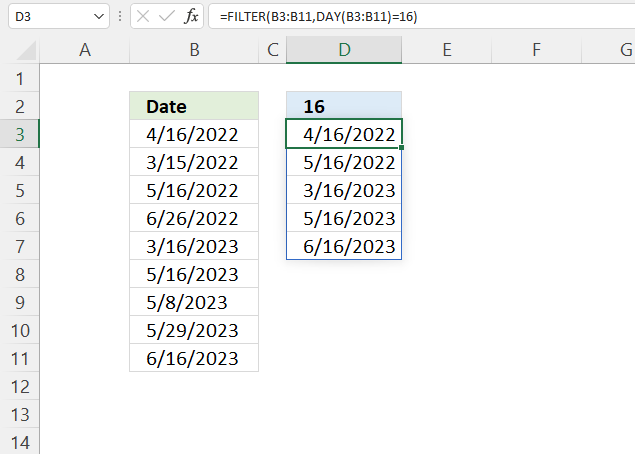Formula in cell D3:

=FILTER(B3:B11,DAY(B3:B11)=16)

### Explaining formula

#### Step 1 - Calculate day number for each date value

DAY(B3:B11)

becomes

DAY({44667; 44667; 44700; 44707; 45006; 45051; 45054; 45075; 45079})

and returns

{16; 15; 16; 26; 16; 16; 8; 29; 16}.

#### Step 2 - Compare month number to condition

The equal sign lets you check if values are equal, note that this does not perform a case-sensitive comparison. Check out the EXACT function if upper and lower letters matter.

DAY(B3:B11)=16

becomes

{16; 15; 16; 26; 16; 16; 8; 29; 16}=16

and returns

{TRUE; FALSE; TRUE; FALSE; TRUE; TRUE; FALSE; FALSE; TRUE}.

#### Step 3 - Extract dates meeting the condition

The FILTER function is a new function available to Excel 365 subscribers. It lets you extract values based on a condition or criteria.

FILTER(arrayinclude, [if_empty])

FILTER(B3:B11, DAY(B3:B11)=16)

becomes

FILTER({44667; 44667; 44700; 44707; 45006; 45051; 45054; 45075; 45079}, {TRUE; FALSE; TRUE; FALSE; TRUE; TRUE; FALSE; FALSE; TRUE})

and returns

{44667; 44700; 45006; 45051; 45079}.

## 7. Create a dynamic calendar using the DAY function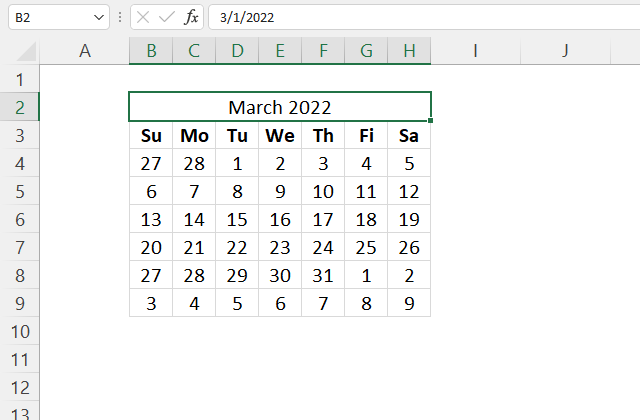The formula in cell B4 is a dynamic array formula and works only in Excel 365. The SEQUENCE function is only available to Excel 365 subscribers.

The formula uses the provided date in cell B2 to calculate all dates for that month. Make sure you only use Excel dates in cell B2 and that the date is the first date in any given month.

The dates in B4:H9 automatically refresh as soon as a new date is entered in cell B2, this is why it is called a dynamic calendar.

Excel 365 formula in cell B4:

=DAY(B2-WEEKDAY(B2,1)+SEQUENCE(6,7))

### Explaining formula

The dynamic array formula in cell B4 is entered as a regular formula, however, it spills values to the right and below as far as needed.

Make sure the adjacent cells are empty or a #SPILL! error is shown.

#### Step 1 - Calculate weekday

This step is needed in order to calculate the first date in the week, it is most often not the same date as the first date in a month. It is the 27th in this example, shown in the image above.

The WEEKDAY function returns a number representing the weekday in a week. 1 - Sunday, 2 - Monday, and so on.

WEEKDAY(serial_number,[return_type])

WEEKDAY(B2,1)

becomes

WEEKDAY(44621, 1)

and returns 3. This means that March the 1st, 2022 is a Tuesday.

#### Step 2 - Create an array large enough to populate a monthly calendar

The SEQUENCE function creates a list of sequential numbers to a cell range or array. It is located in the Math and trigonometry category and is only available to Excel 365 subscribers.

SEQUENCE(rows, [columns], [start], [step])

SEQUENCE(6,7)

returns

{1, 2, 3, 4, 5, 6, 7; 8, 9, 10, 11, 12, 13, 14; 15, 16, 17, 18, 19, 20, 21; 22, 23, 24, 25, 26, 27, 28; 29, 30, 31, 32, 33, 34, 35; 36, 37, 38, 39, 40, 41, 42}.

Note the delimiting characters used in the array above, the comma separates values between columns and the semicolon between rows. Your setup may use other delimiting characters, you can change these in the regional settings.

#### Step 3 - Calculate the date for the first sunday

This is often a date in the previous month. The plus and minus sign lets you perform arithmetic calculations in an Excel formula.

B2-WEEKDAY(B2,1)+SEQUENCE(6,7)

becomes

44621 - 3 + {1, 2, 3, 4, 5, 6, 7; 8, 9, 10, 11, 12, 13, 14; 15, 16, 17, 18, 19, 20, 21; 22, 23, 24, 25, 26, 27, 28; 29, 30, 31, 32, 33, 34, 35; 36, 37, 38, 39, 40, 41, 42}

and returns

{44619, 44620, 44621, 44622, 44623, 44624, 44625; 44626, 44627, 44628, 44629, 44630, 44631, 44632; 44633, 44634, 44635, 44636, 44637, 44638, 44639; 44640, 44641, 44642, 44643, 44644, 44645, 44646; 44647, 44648, 44649, 44650, 44651, 44652, 44653; 44654, 44655, 44656, 44657, 44658, 44659, 44660}

#### Step 4 - Calculate the day for each date in the array

DAY(B2-WEEKDAY(B2,1)+SEQUENCE(6,7))

becomes

DAY({44619, 44620, 44621, 44622, 44623, 44624, 44625; 44626, 44627, 44628, 44629, 44630, 44631, 44632; 44633, 44634, 44635, 44636, 44637, 44638, 44639; 44640, 44641, 44642, 44643, 44644, 44645, 44646; 44647, 44648, 44649, 44650, 44651, 44652, 44653; 44654, 44655, 44656, 44657, 44658, 44659, 44660})

and returns

{27, 28, 1, 2, 3, 4, 5; 6, 7, 8, 9, 10, 11, 12; 13, 14, 15, 16, 17, 18, 19; 20, 21, 22, 23, 24, 25, 26; 27, 28, 29, 30, 31, 1, 2; 3, 4, 5, 6, 7, 8, 9}.